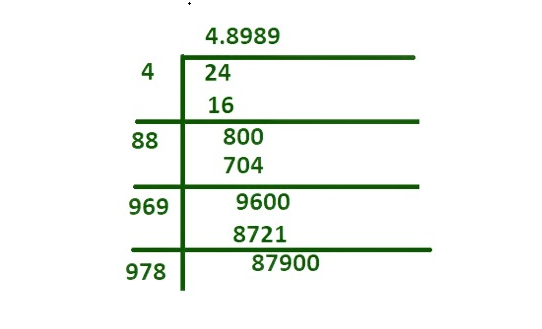Open in App
Not now

# Is the square root of 22 a rational number?

• Last Updated : 01 Jun, 2022

Rational numbers are the numbers that can be expressed as the ratio of two integers. It includes all the integers and can be expressed in terms of fractions or decimals. It is denoted by ‘Q’. When a rational number is divided, the output is in decimal form, which can be either ending or repeating. 3, 4, 5, and so on are some examples of rational numbers as they can be expressed in fraction form as 3/1, 4/1, and 5/1.

Irrational numbers are numbers that cannot be expressed in fractions or ratios of integers. It can be written in decimals and have endless non-repeating digits after the decimal point. It is denoted by ‘P’.

### Square root

The number on which multiplication of a number by itself gives the original number is termed as the square root. The square root of a number is a value that can be written in the form of x = √y. It means ‘x’ is equal to the square root of y, where ‘x’ is any natural number. We can also express it as x2 = y.

Therefore, the square root is a value which when multiplied by itself gives the original number, i.e. y = x × x. Example: 5 × 5 =25, and the square root of 25 is 5. For example,

• Find the square root of 16?

Solution:

Given: √16

= √(4 × 4)

= 4, hence 4 is the square root of 16.

• Find the square root of 24?

Solution:Here square root of 24 is irrational as 4.898979… Hence it is non terminating and non recurring .

### Is the square root of 22 a rational number?

Rational numbers are the numbers that can be expressed as the ratio of two integers. It includes all the integers and can be expressed in terms of fractions or decimals. It is denoted by ‘Q’.

Example: -4 , -6 , -14 , 0 , 1 , 2 , 5 etc

Rational numbers are of the form p/q, where p and q are integers and q ≠ 0. Because of the underlying structure of numbers, p/q form, most individuals find it difficult to distinguish between fractions and rational numbers.

When a rational number is divided, the output is in decimal form, which can be either ending or repeating. 3, 4, 5, and so on are some examples of rational numbers as they can be expressed in fraction form as 3/1, 4/1, and 5/1.

A rational number is a sort of real number that has the form p/q where q≠0. When a rational number is split, the result is a decimal number, which can be either a terminating or a recurring decimal.

Therefore the square root of 22 is √22 = 4.690415….

Here, the answer to above question is Square root of 22 is not a rational number as it is non terminating or not recurring after decimal …

### Similar Problems

Question 1: Determine whether 4.6904…. is a rational number?

Here, the given number, 4.6904…. has not recurring digits.Hence, 4.6904…. is not a rational number.

Question 2: Is the square root of 6 a rational number?

Here, the given number, √6 cannot be expressed in the form of p/q. Alternatively, 6 is not a prime number but a rational number. √6 is equal to 2.449489… which gives the result of non terminating and non recurring digit after decimal, and cannot be expressed as fraction..,

So √6 is not a rational Number .

Question 3: Determine whether -7 is a rational number or an irrational number.

A rational number is a sort of real number that has the form p/q where q≠0. When a rational number is split, the result is a decimal number, which can be either a terminating or a recurring decimal.

Here, the given number -8 is a rational number.

Question 4: Is √2 a rational number?

A rational number is a sort of real number that has the form p/q where q≠0. When a rational number is split, the result is a decimal number, which can be either a terminating or a recurring decimal. Here, the given number, √2 cannot be expressed in the form of p/q. Alternatively, 2 is a prime number or rational number.

Here, the given number √2 is equal to 1.4121 which gives the result of non terminating and non recurring decimal, and cannot be expressed as fraction ..,

So √2 is Irrational Number.

Question 5: Is the square root of 12 a rational or irrational number?

Here, the given number, √12 cannot be expressed in the form of p/q. Alternatively, 12 is not a prime number but a rational number.

Here, the given number √12 is equal to 3.4641016… which gives the result of non terminating and non recurring digit after decimal, and cannot be expressed as fraction .., So √12 is Irrational Number.

Question 6: Is √17 a rational number or an irrational number?